# cwt

Continuous 1-D wavelet transform

See `cwt` for information on the older version of the `cwt`. The older version is no longer recommended.

## Syntax

``wt = cwt(x)``
``wt = cwt(x,wname)``
``````[wt,f] = cwt(___,fs)``````
``````[wt,period] = cwt(___,ts)``````
``````[wt,f,coi] = cwt(___)``````
``````[wt,period,coi] = cwt(___,ts)``````
``[___,coi,fb] = cwt(___)``
``[___,fb,scalingcfs] = cwt(___)``
``[___] = cwt(___,Name=Value)``
``cwt(___)``

## Description

example

````wt = cwt(x)` returns the continuous wavelet transform (CWT) of `x`. The CWT is obtained using the analytic Morse wavelet with the symmetry parameter, gamma ($\gamma$), equal to 3 and the time-bandwidth product equal to 60. `cwt` uses 10 voices per octave. The minimum and maximum scales are determined automatically based on the energy spread of the wavelet in frequency and time.The `cwt` function uses L1 normalization. With L1 normalization, if you have equal amplitude oscillatory components in your data at different scales, they will have equal magnitude in the CWT. Using L1 normalization shows a more accurate representation of the signal. See L1 Norm for CWT and Continuous Wavelet Transform of Two Complex Exponentials.```
````wt = cwt(x,wname)` uses the analytic wavelet specified by `wname` to compute the CWT.```

example

``````[wt,f] = cwt(___,fs)``` specifies the sampling frequency, `fs`, in hertz, and returns the scale-to-frequency conversions `f` in hertz. If you do not specify a sampling frequency, `cwt` returns `f` in cycles per sample.```
``````[wt,period] = cwt(___,ts)``` specifies the sampling period, `ts`, as a positive `duration` scalar. `cwt` uses `ts` to compute the scale-to-period conversions, `period`. `period` is an array of durations with the same Format property as `ts`.```

example

``````[wt,f,coi] = cwt(___)``` returns the cone of influence, `coi`, in cycles per sample. Specify a sampling frequency, `fs`, in hertz, to return the cone of influence in hertz. ```
``````[wt,period,coi] = cwt(___,ts)``` returns the cone of influence, `coi`, as an array of durations with the same Format property as `ts`.```
````[___,coi,fb] = cwt(___)` returns the filter bank used in the CWT. See `cwtfilterbank`.```
````[___,fb,scalingcfs] = cwt(___)` returns the scaling coefficients for the wavelet transform.```
````[___] = cwt(___,Name=Value)` specifies one or more additional name-value arguments. For example, ```wt = cwt(x,TimeBandwidth=40,VoicesPerOctave=20)``` specifies a time-bandwidth product of 40 and 20 voices per octave. ```

example

````cwt(___)` with no output arguments plots the CWT scalogram. The scalogram is the absolute value of the CWT plotted as a function of time and frequency. Frequency is plotted on a logarithmic scale. The cone of influence showing where edge effects become significant is also plotted. Gray regions outside the dashed white line delineate regions where edge effects are significant. If the input signal is complex-valued, the positive (counterclockwise) and negative (clockwise) components are plotted in separate scalograms.If you do not specify a sampling frequency or sampling period, the frequencies are plotted in cycles per sample. If you specify a sampling frequency, the frequencies are in hertz. If you specify a sampling period, the scalogram is plotted as a function of time and periods. If the input signal is a timetable, the scalogram is plotted as a function of time and frequency in hertz and uses the RowTimes as the basis for the time axis.To see the time, frequency, and magnitude of a scalogram point, enable data tips in the figure axes toolbar and click the desired point in the scalogram.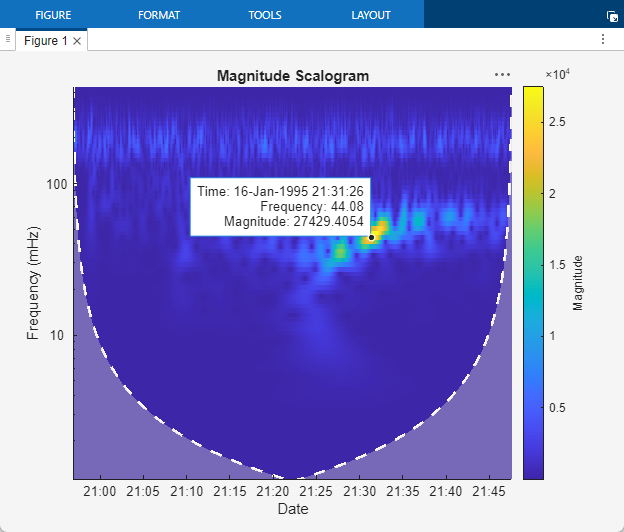Note Before plotting, `cwt` clears (`clf`) the current figure. To plot the scalogram in a subplot, use a plotting function. See Plot CWT Scalogram in Subplot. ```

## Examples

collapse all

Obtain the continuous wavelet transform of a speech sample using default values.

```load mtlb; w = cwt(mtlb);```

`load mtlb`

Loading the file `mtlb.mat` brings the speech signal, `mtlb`, and the sample rate, `Fs`, into the workspace. Display the scalogram of the speech sample obtained using the bump wavelet.

```load mtlb cwt(mtlb,"bump",Fs)```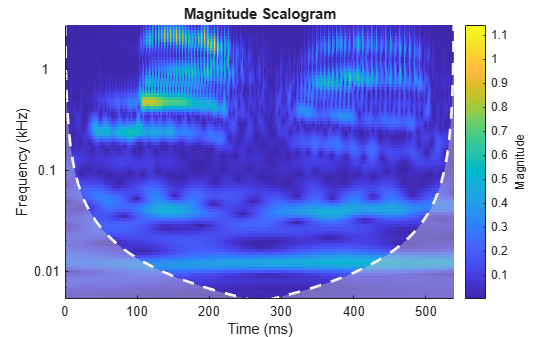Compare with the scalogram obtained using the default Morse wavelet.

`cwt(mtlb,Fs)`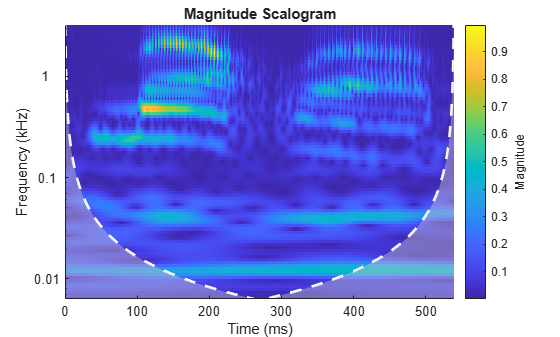Obtain the CWT of the Kobe earthquake data. The data are seismograph (vertical acceleration, nm/sq.sec) measurements recorded at Tasmania University, Hobart, Australia on 16 January 1995 beginning at 20:56:51 (GMT) and continuing for 51 minutes. The sampling frequency is 1 Hz.

`load kobe`

Plot the earthquake data.

```plot((1:numel(kobe))./60,kobe) xlabel("Time (mins)") ylabel("Vertical Acceleration (nm/s^2)") title("Kobe Earthquake Data") grid on axis tight```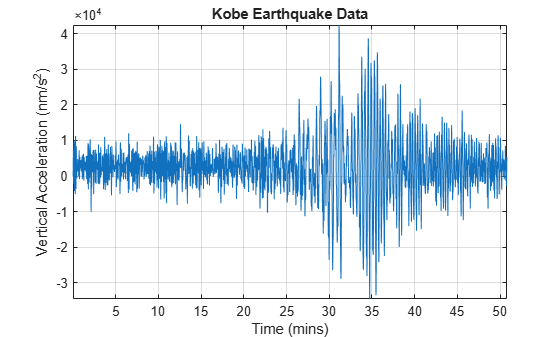Obtain the CWT, frequencies, and cone of influence.

`[wt,f,coi] = cwt(kobe,1);`

View the scalogram, including the cone of influence.

`cwt(kobe,1)`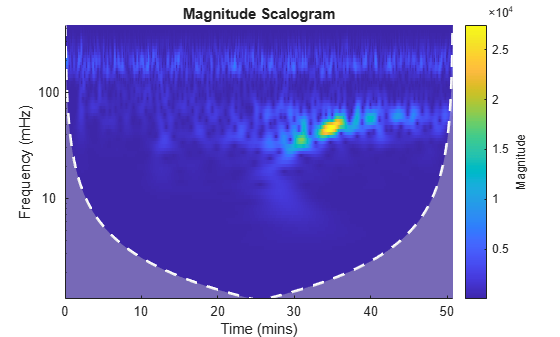Obtain the CWT, time periods, and cone of influence by specifying a sampling period instead of a sampling frequency.

`[wt,periods,coi] = cwt(kobe,minutes(1/60));`

View the scalogram generated when specifying a sampling period.

`cwt(kobe,minutes(1/60))`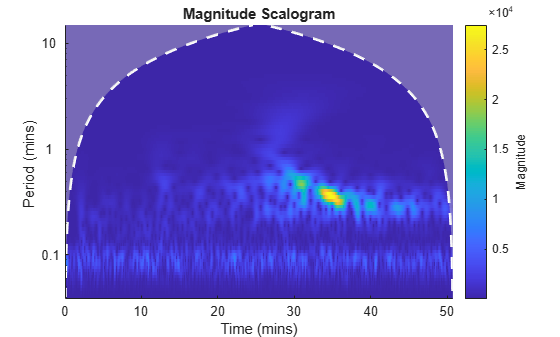Create two complex exponentials, of different amplitudes, with frequencies of 32 and 64 Hz. The data is sampled at 1000 Hz. The two complex exponentials have disjoint support in time.

```Fs = 1e3; t = 0:1/Fs:1; z = exp(1i*2*pi*32*t).*(t>=0.1 & t<0.3)+2*exp(-1i*2*pi*64*t).*(t>0.7);```

Add complex white Gaussian noise with a standard deviation of 0.05.

```wgnNoise = 0.05/sqrt(2)*randn(size(t))+1i*0.05/sqrt(2)*randn(size(t)); z = z+wgnNoise;```

Obtain and plot the cwt using a Morse wavelet.

`cwt(z,Fs)`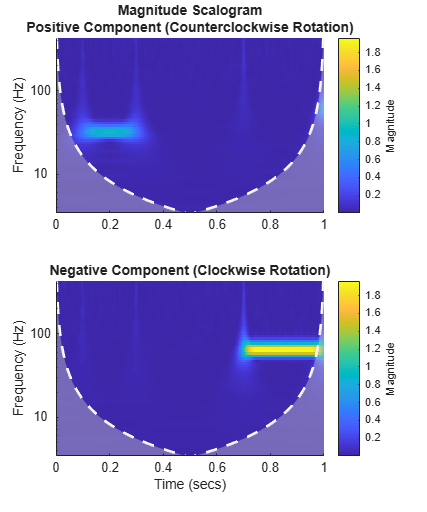Note the magnitudes of the complex exponential components in the colorbar are essentially their amplitudes even though they are at different scales. This is a direct result of the L1 normalization. You can verify this by executing this script and exploring each subplot with a data cursor.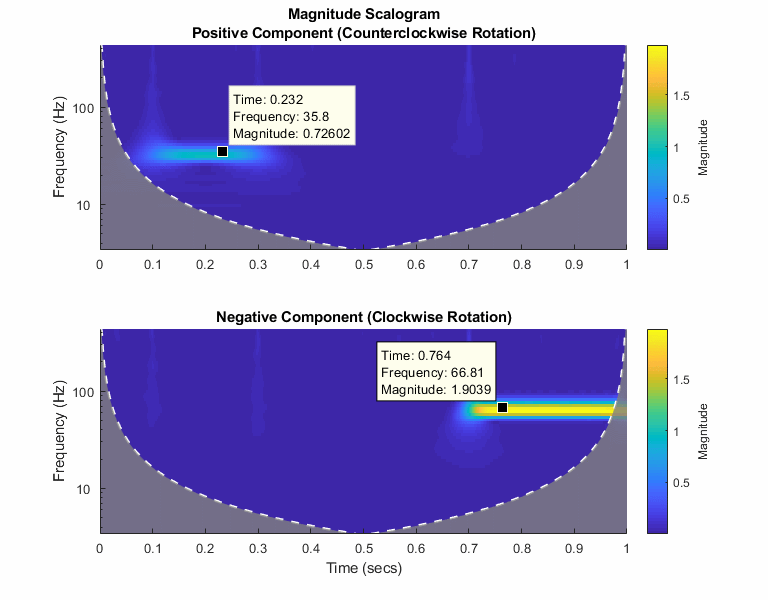This example shows that the amplitudes of oscillatory components in a signal agree with the amplitudes of the corresponding wavelet coefficients.

Create a signal composed of two sinusoids with disjoint support in time. One sinusoid has a frequency of 32 Hz and amplitude equal to 1. The other sinusoid has a frequency of 64 Hz and amplitude equal to 2. The signal is sampled for one second at 1000 Hz. Plot the signal.

```frq1 = 32; amp1 = 1; frq2 = 64; amp2 = 2; Fs = 1e3; t = 0:1/Fs:1; x = amp1*sin(2*pi*frq1*t).*(t>=0.1 & t<0.3)+... amp2*sin(2*pi*frq2*t).*(t>0.6 & t<0.9); plot(t,x) grid on xlabel("Time (sec)") ylabel("Amplitude") title("Signal")```Create a CWT filter bank that can be applied to the signal. Since the signal component frequencies are known, set the frequency limits of the filter bank to a narrow range that includes the known frequencies. To confirm the range, plot the magnitude frequency responses for the filter bank.

```fb = cwtfilterbank(SignalLength=numel(x),SamplingFrequency=Fs,... FrequencyLimits=[20 100]); freqz(fb)```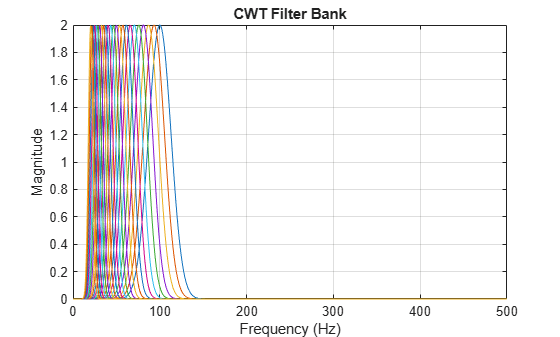Use `cwt` and the filter bank to plot the scalogram of the signal.

`cwt(x,FilterBank=fb)`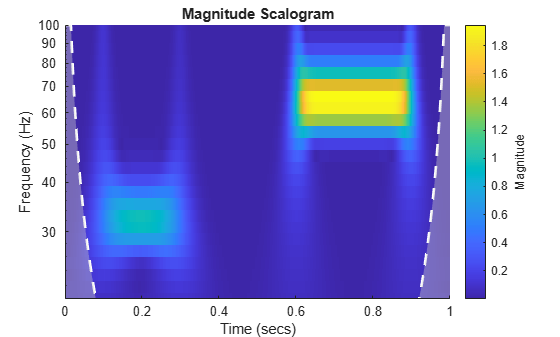Use a data cursor to confirm that the amplitudes of the wavelet coefficients are essentially equal to the amplitudes of the sinusoidal components. Your results should be similar to the ones in the following figure.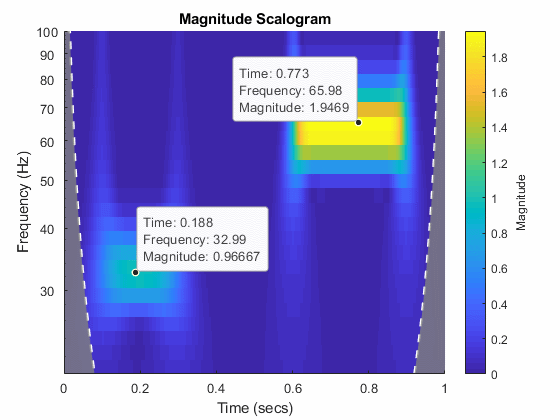This example shows how using a CWT filter bank can improve computational efficiency when taking the CWT of multiple time series.

Create a 100-by-1024 matrix `x`. Create a CWT filter bank appropriate for signals with 1024 samples.

```x = randn(100,1024); fb = cwtfilterbank;```

Use `cwt` with default settings to obtain the CWT of a signal with 1024 samples. Create a 3-D array that can contain the CWT coefficients of 100 signals, each of which has 1024 samples.

```cfs = cwt(x(1,:)); res = zeros(100,size(cfs,1),size(cfs,2));```

Use the `cwt` function and take the CWT of each row of the matrix `x`. Display the elapsed time.

```tic for k=1:100 res(k,:,:) = cwt(x(k,:)); end toc```
```Elapsed time is 0.928160 seconds. ```

Now use the `wt` object function of the filter bank to take the CWT of each row of `x`. Display the elapsed time.

```tic for k=1:100 res(k,:,:) = wt(fb,x(k,:)); end toc```
```Elapsed time is 0.393524 seconds. ```

This example shows how to generate a MEX file to perform the continuous wavelet transform (CWT) using generated CUDA code.

First, ensure that you have a CUDA-enabled GPU and the NVCC compiler. See The GPU Environment Check and Setup App (GPU Coder) to ensure you have the proper configuration.

Create a GPU coder configuration object.

`cfg = coder.gpuConfig("mex");`

Generate a signal of 100,000 samples at 1,000 Hz. The signal consists of two cosine waves with disjoint time supports.

```t = 0:.001:(1e5*0.001)-0.001; x = cos(2*pi*32*t).*(t > 10 & t<=50)+ ... cos(2*pi*64*t).*(t >= 60 & t < 90)+ ... 0.2*randn(size(t));```

Cast the signal to use single precision. GPU calculations are often more efficiently done in single precision. You can however also generate code for double precision if your NVIDIA GPU supports it.

`x = single(x);`

Generate the GPU MEX file and a code generation report. To allow generation of the MEX file, you must specify the properties (class, size, and complexity) of the three input parameters:

• `coder.typeof(single(0),[1 1e5])` specifies a row vector of length 100,000 containing real `single` values.

• `coder.typeof('c',[1 inf])` specifies a character array of arbitrary length.

• `coder.typeof(0)` specifies a real `double` value.

```sig = coder.typeof(single(0),[1 1e5]); wav = coder.typeof('c',[1 inf]); sfrq = coder.typeof(0); codegen cwt -config cfg -args {sig,wav,sfrq} -report```
```Code generation successful: View report ```

The -report flag is optional. Using `-report` generates a code generation report. In the Summary tab of the report, you can find a GPU code metrics link, which provides detailed information such as the number of CUDA kernels generated and how much memory was allocated.

Run the MEX file on the data and plot the scalogram. Confirm the plot is consistent with the two disjoint cosine waves.

```[cfs,f] = cwt_mex(x,'morse',1e3); image("XData",t,"YData",f,"CData",abs(cfs),"CDataMapping","scaled") set(gca,"YScale","log") axis tight xlabel("Time (Seconds)") ylabel("Frequency (Hz)") title("Scalogram of Two-Tone Signal")```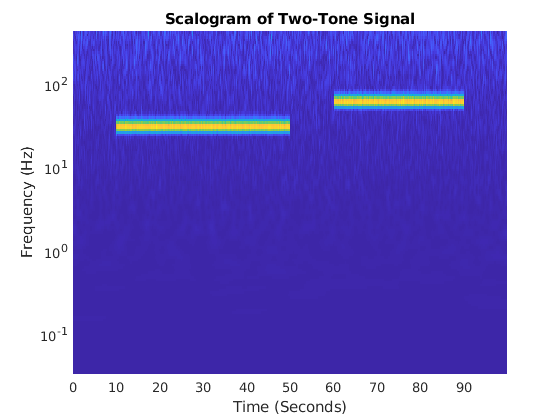Run the CWT command above without appending the `_``mex`. Confirm the MATLAB and the GPU MEX scalograms are identical.

```[cfs2,f2] = cwt(x,'morse',1e3); max(abs(cfs2(:)-cfs(:)))```
```ans = single 7.3380e-07 ```

This example shows how to change the default frequency axis labels for the CWT when you obtain a plot with no output arguments.

Create two sine waves with frequencies of 32 and 64 Hz. The data is sampled at 1000 Hz. The two sine waves have disjoint support in time. Add white Gaussian noise with a standard deviation of 0.05. Obtain and plot the CWT using the default Morse wavelet.

```Fs = 1e3; t = 0:1/Fs:1; x = cos(2*pi*32*t).*(t>=0.1 & t<0.3)+sin(2*pi*64*t).*(t>0.7); wgnNoise = 0.05*randn(size(t)); x = x+wgnNoise; cwt(x,1000)```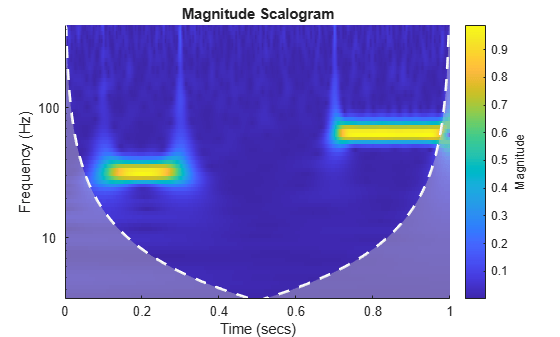The plot uses a logarithmic frequency axis because frequencies in the CWT are logarithmic. In MATLAB, logarithmic axes are in powers of 10 (decades). You can use `cwtfreqbounds` to determine what the minimum and maximum wavelet bandpass frequencies are for a given signal length, sampling frequency, and wavelet.

`[minf,maxf] = cwtfreqbounds(numel(x),1000);`

You see that by default MATLAB has placed frequency ticks at 10 and 100 because those are the powers of 10 between the minimum and maximum frequencies. If you wish to add more frequency axis ticks, you can obtain a logarithmically spaced set of frequencies between the minimum and maximum frequencies using the following.

```numfreq = 10; freq = logspace(log10(minf),log10(maxf),numfreq);```

Next, get the handle to the current axes and replace the frequency axis ticks and labels with the following.

```AX = gca; AX.YTickLabelMode = "auto"; AX.YTick = freq;```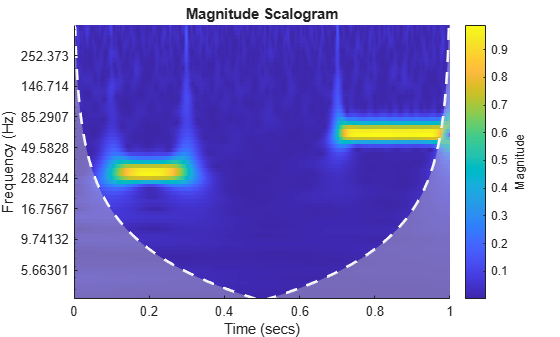In the CWT, frequencies are computed in powers of two. To create the frequency ticks and tick labels in powers of two, you can do the following.

```newplot cwt(x,1000) AX = gca; freq = 2.^(round(log2(minf)):round(log2(maxf))); AX.YTickLabelMode = "auto"; AX.YTick = freq;```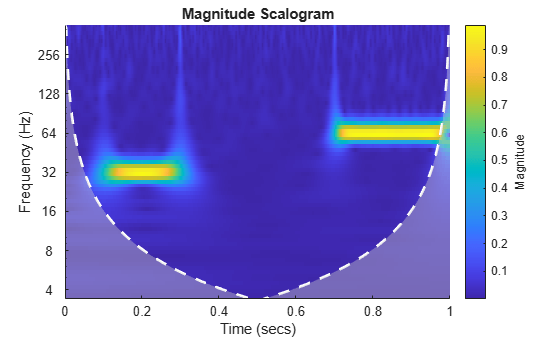This example shows how to scale scalogram values by maximum absolute value at each level for plotting.

Load in a signal and display the default scalogram. Change the colormap to `pink(240)`.

```load noisdopp cwt(noisdopp) colormap(pink(240))```Take the CWT of the signal and obtain the wavelet coefficients and frequencies.

`[cfs,frq] = cwt(noisdopp);`

To efficiently find the maximum value of the coefficients at each frequency (level), first transpose the absolute value of the coefficients. Find the minimum value at every level. At each level, subtract the level's minimum value.

```tmp1 = abs(cfs); t1 = size(tmp1,2); tmp1 = tmp1'; minv = min(tmp1); tmp1 = (tmp1-minv(ones(1,t1),:));```

Find the maximum value at every level of `tmp1`. For each level, divide every value by the maximum value at that level. Multiply the result by the number of colors in the colormap. Set equal to 1 all zero entries. Transpose the result.

```maxv = max(tmp1); maxvArray = maxv(ones(1,t1),:); indx = maxvArray<eps; tmp1 = 240*(tmp1./maxvArray); tmp2 = 1+fix(tmp1); tmp2(indx) = 1; tmp2 = tmp2';```

Display the result. The scalogram values are now scaled by the maximum absolute value at each level. Frequencies are displayed on a linear scale.

```t = 0:length(noisdopp)-1; pcolor(t,frq,tmp2) shading interp xlabel("Time (Samples)") ylabel("Normalized Frequency (cycles/sample)") title("Scalogram Scaled By Level") colormap(pink(240)) colorbar```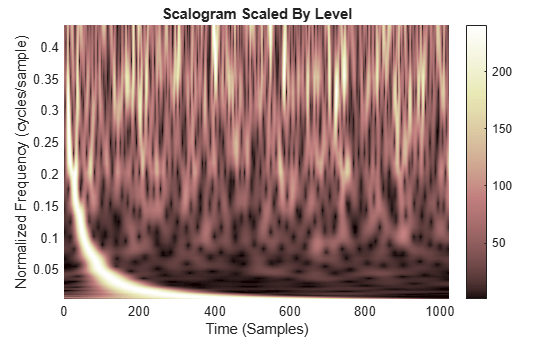This example shows that increasing the time-bandwidth product ${P}^{2}$ of the Morse wavelet creates a wavelet with more oscillations under its envelope. Increasing ${P}^{2}$ narrows the wavelet in frequency.

Create two filter banks. One filter bank has the default `TimeBandwidth` value of 60. The second filter bank has a `TimeBandwidth` value of 10. The `SignalLength` for both filter banks is 4096 samples.

```sigLen = 4096; fb60 = cwtfilterbank(SignalLength=sigLen); fb10 = cwtfilterbank(SignalLength=sigLen,TimeBandwidth=10);```

Obtain the time-domain wavelets for the filter banks.

```[psi60,t] = wavelets(fb60); [psi10,~] = wavelets(fb10);```

Use the `scales` function to find the mother wavelet for each filter bank.

```sca60 = scales(fb60); sca10 = scales(fb10); [~,idx60] = min(abs(sca60-1)); [~,idx10] = min(abs(sca10-1)); m60 = psi60(idx60,:); m10 = psi10(idx10,:);```

Since the time-bandwidth product is larger for the `fb60` filter bank, verify the `m60` wavelet has more oscillations under its envelope than the `m10` wavelet.

```subplot(2,1,1) plot(t,abs(m60)) grid on hold on plot(t,real(m60)) plot(t,imag(m60)) hold off xlim([-30 30]) legend("abs(m60)","real(m60)","imag(m60)") title("TimeBandwidth = 60") subplot(2,1,2) plot(t,abs(m10)) grid on hold on plot(t,real(m10)) plot(t,imag(m10)) hold off xlim([-30 30]) legend("abs(m10)","real(m10)","imag(m10)") title("TimeBandwidth = 10")```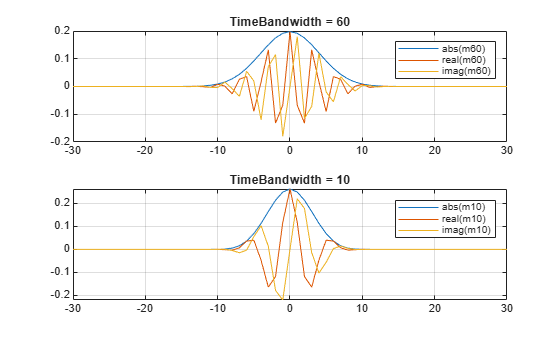Align the peaks of the `m60` and `m10` magnitude frequency responses. Verify the frequency response of the `m60` wavelet is narrower than the frequency response for the `m10` wavelet.

```cf60 = centerFrequencies(fb60); cf10 = centerFrequencies(fb10); m60cFreq = cf60(idx60); m10cFreq = cf10(idx10); freqShift = 2*pi*(m60cFreq-m10cFreq); x10 = m10.*exp(1j*freqShift*(-sigLen/2:sigLen/2-1)); figure plot([abs(fft(m60)).' abs(fft(x10)).']) grid on legend("Time-bandwidth = 60","Time-bandwidth = 10") title("Magnitude Frequency Responses")```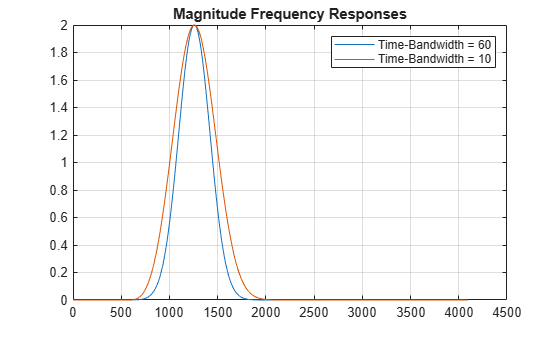This example shows how to plot the CWT scalogram in a figure subplot.

Load the speech sample. The data is sampled at 7418 Hz. Plot the default CWT scalogram.

```load mtlb cwt(mtlb,Fs)```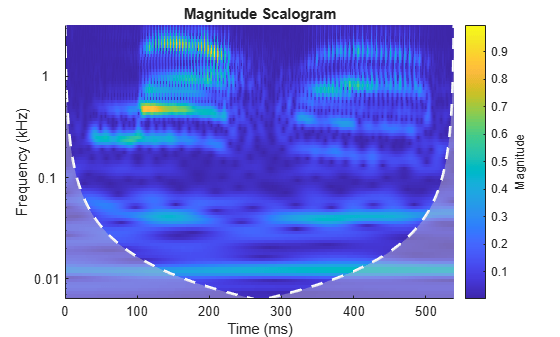Obtain the continuous wavelet transform of the signal, and the frequencies of the CWT.

`[cfs,frq] = cwt(mtlb,Fs);`

The `cwt` function sets the time and frequency axes in the scalogram. Create a vector representing the sample times.

`tms = (0:numel(mtlb)-1)/Fs;`

In a new figure, plot the original signal in the upper subplot and the scalogram in the lower subplot. Plot the frequencies on a logarithmic scale.

```figure subplot(2,1,1) plot(tms,mtlb) axis tight title("Signal and Scalogram") xlabel("Time (s)") ylabel("Amplitude") subplot(2,1,2) surface(tms,frq,abs(cfs)) axis tight shading flat xlabel("Time (s)") ylabel("Frequency (Hz)") set(gca,"yscale","log")```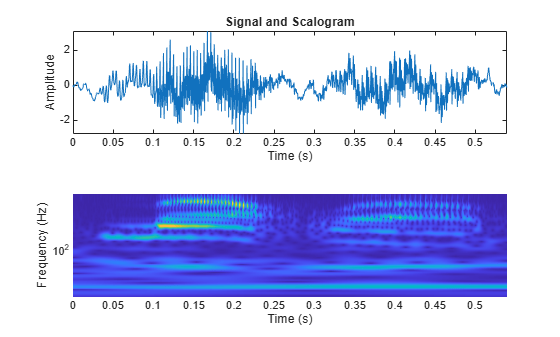## Input Arguments

collapse all

Input signal, specified as a real- or complex-valued vector, or a single-variable regularly sampled timetable. The input `x` must have at least four samples.

The `cwt` function also accepts GPU array inputs. For more information, see Run MATLAB Functions on a GPU (Parallel Computing Toolbox).

Data Types: `single` | `double`
Complex Number Support: Yes

Analytic wavelet used to compute the CWT. Valid options for `wname` are `"morse"`, `"amor"`, and `"bump"`, which specify the Morse, Morlet (Gabor), and bump wavelet, respectively.

The default Morse wavelet has symmetry parameter gamma ($\gamma$) equal to 3 and time-bandwidth product equal to 60.

Data Types: `char` | `string`

Sampling frequency in hertz, specified as a positive scalar. If you specify `fs`, then you cannot specify `ts`. If `x` is a timetable, you cannot specify `fs`. `fs` is determined from the RowTimes of the timetable.

Data Types: `single` | `double`

Sampling period, also known as the time duration, specified as a scalar duration. Valid durations are `years`, `days`, `hours`, `minutes`, and `seconds`. You cannot use calendar durations. If you specify `ts`, then you cannot specify `fs`. If `x` is a timetable, you cannot specify `ts`. `ts` is determined from the RowTimes of the timetable when you set the `PeriodLimits` name-value argument.

Example: `wt = cwt(x,hours(12))`

Data Types: `duration`

### Name-Value Arguments

Specify optional pairs of arguments as `Name1=Value1,...,NameN=ValueN`, where `Name` is the argument name and `Value` is the corresponding value. Name-value arguments must appear after other arguments, but the order of the pairs does not matter.

Example: `wt = cwt(x,"bump",VoicesPerOctave=10)` returns the CWT of `x` using the bump wavelet and 10 voices per octave.

Before R2021a, use commas to separate each name and value, and enclose `Name` in quotes.

Example: ```wt = cwt(x,"ExtendedSignal",true,"FrequencyLimits",[0.1 0.2])``` extends the input signal symmetrically and specifies frequency limits of 0.1 to 0.2 samples per cycle.

Option to extend the input signal symmetrically by reflection, specified as one of these:

• `1` (`true`) — Extend symmetrically

• `0` (`false`) — Do not extend symmetrically

If `ExtendSignal` is `false`, the signal is extended periodically. Extending the signal symmetrically can mitigate boundary effects.

Note

If you want to invert the CWT using `icwt` with scaling coefficients and approximate synthesis filters, set `ExtendSignal` to `false`.

Data Types: `logical`

Frequency limits to use in the CWT, specified as a two-element vector with positive strictly increasing entries. The first element specifies the lowest peak passband frequency and must be greater than or equal to the product of the wavelet peak frequency in hertz and two time standard deviations divided by the signal length. The second element specifies the highest peak passband frequency and must be less than or equal to the Nyquist frequency. The base-2 logarithm of the ratio of the upper frequency limit, `freqMax`, to the lower frequency limit, `freqMin`, must be greater than or equal to `1/NV`, where `NV` is the number of voices per octave:

```log2(freqMax/freqMin) ≥ 1/NV```.

If you specify frequency limits outside the permissible range, `cwt` truncates the limits to the minimum and maximum valid values. Use `cwtfreqbounds` to determine frequency limits for different parameterizations of the CWT. For complex-valued signals, (-1) × `flimits` is used for the anti-analytic part, where `flimits` is the vector specified by `FrequencyLimits`.

Example: ```wt = cwt(x,1000,VoicesPerOctave=10,FrequencyLimits=[80 90])```

Data Types: `double`

Period limits to use in the CWT, specified as a two-element duration array with strictly increasing positive entries. The first element must be greater than or equal to 2 × `ts` where `ts` is the sampling period. The maximum period cannot exceed the signal length divided by the product of two time standard deviations of the wavelet and the wavelet peak frequency. The base-2 logarithm of the ratio of the minimum period, `minP`, to the maximum period, `maxP`, must be less than or equal to `-1/NV`, where `NV` is the number of voices per octave:

```log2(pMin/pMax) ≤ -1/NV```.

If you specify period limits outside the permissible range, `cwt` truncates the limits to the minimum and maximum valid values. Use `cwtfreqbounds` to determine period limits for different parameterizations of the wavelet transform. For complex-valued signals, (-1) × `plimits` is used for the anti-analytic part, where `plimits` is the vector specified by `PeriodLimits`.

Example: ```wt = cwt(x,seconds(0.1),VoicesPerOctave=10,PeriodLimits=[seconds(0.2) seconds(3)])```

Data Types: `duration`

Number of voices per octave to use for the CWT, specified as an integer from 1 to 48. The CWT scales are discretized using the specified number of voices per octave. The energy spread of the wavelet in frequency and time automatically determines the minimum and maximum scales.

Time-bandwidth product of the Morse wavelet, specified as a scalar greater than or equal to 3 and less than or equal to 120. The symmetry parameter, gamma ($\gamma$), is fixed at 3. Wavelets with larger time-bandwidth products have larger spreads in time and narrower spreads in frequency. The standard deviation of the Morse wavelet in time is approximately `sqrt(TimeBandwidth/2)`. The standard deviation of the Morse wavelet in frequency is approximately ```1/2 × sqrt(2/TimeBandwidth)```.

If you specify `TimeBandwidth`, you cannot specify `WaveletParameters`. To specify both the symmetry and time-bandwidth product, use `WaveletParameters` instead.

In the notation of Morse Wavelets, `TimeBandwidth` is P2.

Symmetry and time-bandwidth product of the Morse wavelet, specified as a two-element vector of scalars. The first element is the symmetry, $\gamma$, which must be greater than or equal to 1. The second element is the time-bandwidth product, which must be greater than or equal to $\gamma$. The ratio of the time-bandwidth product to $\gamma$ cannot exceed 40.

When $\gamma$ is equal to 3, the Morse wavelet is perfectly symmetric in the frequency domain and the skewness is 0. When $\gamma$ is greater than 3, the skewness is positive. When $\gamma$ is less than 3, the skewness is negative.

If you specify `WaveletParameters`, you cannot specify `TimeBandwidth`.

CWT filter bank to use to compute the CWT, specified as a `cwtfilterbank` object. If you set `FilterBank`, you cannot specify any other options. All options for the computation of the CWT are defined as properties of the filter bank. For more information, see `cwtfilterbank`.

If `x` is a timetable, the sampling frequency or sampling period in `fb` must agree with the sampling frequency or sampling period determined by the `RowTimes` of the timetable.

Example: `wt = cwt(x,FilterBank=cfb)`

## Output Arguments

collapse all

Continuous wavelet transform, returned as a matrix of complex values. By default, `cwt` uses the analytic Morse (3,60) wavelet, where 3 is the symmetry and 60 is the time-bandwidth product. `cwt` uses 10 voices per octave.

• If `x` is real-valued, `wt` is an Na-by-N matrix, where Na is the number of scales, and N is the number of samples in `x`.

• If `x` is complex-valued, `wt` is a 3-D matrix, where the first page is the CWT for the positive scales (analytic part or counterclockwise component) and the second page is the CWT for the negative scales (anti-analytic part or clockwise component).

The minimum and maximum scales are determined automatically based on the energy spread of the wavelet in frequency and time. See Algorithms for information on how the scales are determined.

Data Types: `single` | `double`

Scale-to-frequency conversions of the CWT, returned as a vector. If you specify a sampling frequency, `fs`, then `f` is in hertz. If you do not specify `fs`, `cwt` returns `f` in cycles per sample. If the input `x` is complex, the scale-to-frequency conversions apply to both pages of `wt`.

Scale-to-period conversions, returned as an array of durations with the same Format property as `ts`. Each row corresponds to a period. If the input `x` is complex, the scale-to-period conversions apply to both pages of `wt`.

Cone of influence for the CWT. If you specify a sampling frequency, `fs`, the cone of influence is in hertz. If you specify a scalar duration, `ts`, the cone of influence is an array of durations with the same Format property as `ts`. If the input `x` is complex, the cone of influence applies to both pages of `wt`.

The cone of influence indicates where edge effects occur in the CWT. Due to the edge effects, give less credence to areas that are outside or overlap the cone of influence. For additional information, see Boundary Effects and the Cone of Influence.

CWT filter bank used in the CWT, returned as a `cwtfilterbank` object. See `cwtfilterbank`.

Scaling coefficients for the CWT, returned as a real- or complex-valued vector. The length of `scalingcfs` is equal to the length of the input `x`.

collapse all

### Analytic Wavelets

Analytic wavelets are complex-valued wavelets whose Fourier transform vanish for negative frequencies. Analytic wavelets are a good choice when doing time-frequency analysis with the CWT. Because the wavelet coefficients are complex-valued, the coefficients provide phase and amplitude information of the signal being analyzed. Analytic wavelets are well suited for studying how the frequency content in real world nonstationary signals evolves as a function of time.

Analytic wavelets are almost exclusively based on rapidly decreasing functions. If $\psi \left(t\right)$ is an analytic rapidly decreasing function in time, then its Fourier transform $\stackrel{^}{\psi }\left(\omega \right)$ is a rapidly decreasing function in frequency and is small outside of some interval $\alpha <\omega <\beta$ where $0<\alpha <\beta$. Orthogonal and biorthogonal wavelets are typically designed to have compact support in time. Wavelets with compact support in time have relatively poorer energy concentration in frequency than wavelets which rapidly decrease in time. Most orthogonal and biorthogonal wavelets are not symmetric in the Fourier domain.

If your goal is to obtain a joint time-frequency representation of your signal, we recommend you use `cwt` or `cwtfilterbank`. Both functions support the following analytic wavelets:

• Morse Wavelet Family (default)

• Analytic Morlet (Gabor) Wavelet

• Bump

If you want to do time-frequency analysis using orthogonal or biorthogonal wavelets, we recommend `modwpt`.

When using wavelets for time-frequency analysis, you usually convert scales to frequencies or periods to interpret results. `cwt` and `cwtfilterbank` do the conversion. You can obtain the corresponding scales associated by using `scales` on the optional `cwt` output argument `fb`.

For more information regarding Morse wavelets, see Morse Wavelets. For guidance on how to choose the wavelet that is right for your application, see Choose a Wavelet.

## Algorithms

collapse all

### Minimum Scale

To determine the minimum scale, find the peak frequency ${\omega }_{x}$ of the base wavelet. For Morse wavelets, dilate the wavelet so that the Fourier transform of the wavelet at $\pi$ radians is equal to 10% of the peak frequency. The smallest scale occurs at the largest frequency:

`${s}_{0}=\frac{{\omega }_{x}^{\text{'}}}{\pi }$`

As a result, the smallest scale is the minimum of (2, ${s}_{0}$). For Morse wavelets, the smallest scale is usually ${s}_{0}$. For the Morlet wavelet, the smallest scale is usually 2.

### Maximum Scale

Both the minimum and maximum scales of the CWT are determined automatically based on the energy spread of the wavelet in frequency and time. To determine the maximum scale, CWT uses the following algorithm.

The standard deviation of the Morse wavelet in time, ${\sigma }_{t}$, is approximately $\sqrt{\frac{{P}^{2}}{2}}$, where ${P}^{2}$ is the time-bandwidth product. The standard deviation in frequency, ${\sigma }_{f}$, is approximately $\frac{1}{2}\sqrt{\frac{2}{{P}^{2}}}$. If you scale the wavelet by some $s>1$, the time duration changes to $2s{\sigma }_{t}=N$, which is the wavelet stretched to equal the full length (N samples) of the input. You cannot translate this wavelet or stretch it further without causing it to wrap, so the largest scale is $floor\left(\frac{N}{2{\sigma }_{t}}\right)$.

Wavelet transform scales are powers of 2 and are denoted by ${s}_{0}{\left({2}^{\frac{1}{NV}}\right)}^{j}$. NV is the number of voices per octave, and j ranges from 0 to the largest scale. For a specific small scale, ${s}_{0}$:

`${s}_{0}{\left({2}^{\frac{1}{NV}}\right)}^{j}\le \frac{N}{2{\sigma }_{t}}$`

Converting to log2:

`$j{\mathrm{log}}_{2}\left({2}^{\frac{1}{NV}}\right)\le {\mathrm{log}}_{2}\left(\frac{N}{2{\sigma }_{t}{s}_{0}}\right)$`

`$j\le NV{\mathrm{log}}_{2}\left(\frac{N}{2{\sigma }_{t}{s}_{0}}\right)$`

Therefore, the maximum scale is

`${s}_{0}{\left({2}^{\frac{1}{NV}}\right)}^{floor\left(NV{\mathrm{log}}_{2}\left(\frac{N}{2{\sigma }_{t}{s}_{0}}\right)\right)}$`

### L1 Norm for CWT

In integral form, the CWT preserves energy. However, when you implement the CWT numerically, energy is not preserved. In this case, regardless of the normalization you use, the CWT is not an orthonormal transform. The `cwt` function uses L1 normalization.

Wavelet transforms commonly use L2 normalization of the wavelet. For the L2 norm, dilating a signal by 1/s, where s is greater than 0, is defined as follows:

`${‖x\left(\frac{t}{s}\right)‖}_{2}^{2}=s{‖x\left(t\right)‖}_{2}^{2}$`

The energy is now s times the original energy. When included in the Fourier transform, multiplying by $1}{\sqrt{s}}$ produces different weights being applied to different scales, so that the peaks at higher frequencies are reduced more than the peaks at lower frequencies.

In many applications, L1 normalization is better. The L1 norm definition does not include squaring the value, so the preserving factor is 1/s instead of $1}{\sqrt{s}}$. Instead of high-frequency amplitudes being reduced as in the L2 norm, for L1 normalization, all frequency amplitudes are normalized to the same value. Therefore, using the L1 norm shows a more accurate representation of the signal. See example Continuous Wavelet Transform of Two Complex Exponentials.

 Lilly, J. M., and S. C. Olhede. “Generalized Morse Wavelets as a Superfamily of Analytic Wavelets.” IEEE Transactions on Signal Processing 60, no. 11 (November 2012): 6036–6041. https://doi.org/10.1109/TSP.2012.2210890.

 Lilly, J.M., and S.C. Olhede. “Higher-Order Properties of Analytic Wavelets.” IEEE Transactions on Signal Processing 57, no. 1 (January 2009): 146–160. https://doi.org/10.1109/TSP.2008.2007607.

 Lilly, J. M. jLab: A data analysis package for Matlab, version 1.6.2. 2016. http://www.jmlilly.net/jmlsoft.html.

 Lilly, Jonathan M. “Element Analysis: A Wavelet-Based Method for Analysing Time-Localized Events in Noisy Time Series.” Proceedings of the Royal Society A: Mathematical, Physical and Engineering Sciences 473, no. 2200 (April 30, 2017): 20160776. https://doi.org/10.1098/rspa.2016.0776.